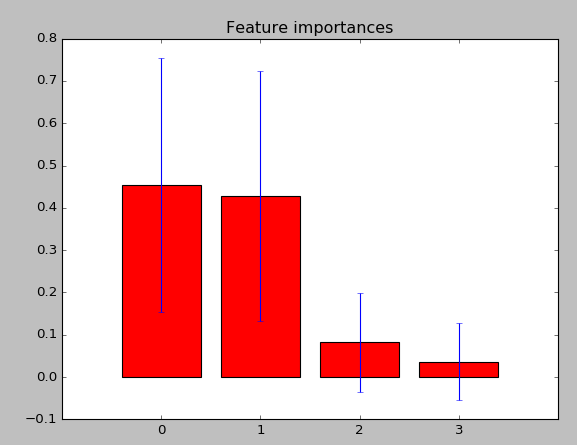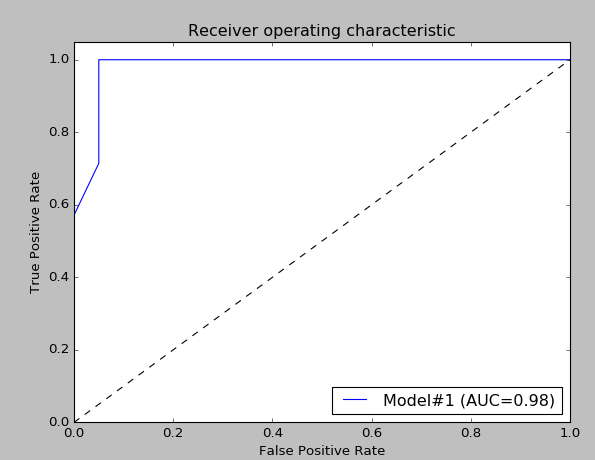# Random Forest Classifier with Python

Random forests is a notion of the general technique of random decision forests that are an ensemble learning method for classification, regression and other tasks, that operate by constructing a multitude of decision trees at training time and outputting the class that is the mode of the classes (classification) or mean prediction (regression) of the individual trees. 

Random Forest models have risen significantly in their popularity – and for some real good reasons. They can be applied quickly to any data science problems to get first set of benchmark results. They are incredibly powerful and can be implemented quickly out of the box. 

In this post we will show implementation of Random Forest algorithm with python. In the script will be shown different functions available in sklearn library for classification. It will be shown how to input data from text file, how to create Random Forest, how to make Receiver Operating Characteristic (ROC) plot and how to create plot for feature importance.

We will use Iris flower dataset to verify the effectiveness of a Random Forest. The Iris flower dataset is widely used in machine learning to test classification techniques. The dataset consists of four measurements taken from each of three species of Iris.
The inputted data have first row as the header row with the following column names: class, petal_length,petal_width,sepal_length,sepal_width. So the first column is Y column and the other 4 columns are X columns. This data format was used in .

You can run online Random Forest at Online Machine Learning Algorithms
Below is the source code for Random Forest. The code was built based on documentation for sklearn library and other examples over the web, references are shown in the end of post. Feel free to post any comment or question related to this topic.

``````import pandas as pd
import numpy as np
import matplotlib.pyplot as plt

from sklearn.metrics import roc_curve, auc
from sklearn.cross_validation import train_test_split
from sklearn.preprocessing import label_binarize
from sklearn.ensemble import RandomForestClassifier

def RF(trainfile, testfile):

cols = ['petal_length', 'petal_width', 'sepal_length', 'sepal_width']
colsRes = ['class']
trainArr_All = train.as_matrix(cols)    # training array
trainRes_All = train.as_matrix(colsRes) # training results

rf = RandomForestClassifier(n_estimators=100)    # 100 decision trees

# Split data train/test = 75/25
trainArr, Xtest, trainRes, ytest = train_test_split(trainArr_All, trainRes_All, test_size=0.25,
random_state=42)

rf.fit(trainArr, trainRes.ravel())

importances = rf.feature_importances_
std = np.std([tree.feature_importances_ for tree in rf.estimators_],
axis=0)
indices = np.argsort(importances)[::-1]

# Print the feature ranking
print("Feature ranking:")

for f in range(trainArr.shape):
print("%d. feature %d (%f)" % (f + 1, indices[f], importances[indices[f]]))

ypred = rf.predict_proba(Xtest)

print ("\nXtest\n")
print (Xtest)
print ("\nytest\n")
print (ytest)
print ("\nypred [:,1]\n")
print (ypred[:,1])
print ("\nypred\n")
print (ypred)
print ("\nClass Labels\n")
print (rf.classes_)

# Binarize ytest data for building roc plot
ytestB = label_binarize(ytest, classes=rf.classes_)

fpr, tpr, threshold = roc_curve(ytestB[:,1], ypred[:, 1])

roc_auc = auc(fpr, tpr)
plt.plot(fpr, tpr, label="Model#%d (AUC=%.2f)" % (1, roc_auc))

# Plot Receiver operating characteristic (ROC)
plt.plot([0, 1], [0, 1], 'k--')
plt.xlim([0.0, 1.0])
plt.ylim([0.0, 1.05])
plt.xlabel('False Positive Rate')
plt.ylabel('True Positive Rate')
plt.legend(loc="lower right")
plt.show()

# Plot the feature importances of the forest
plt.figure()
plt.title("Feature importances")
plt.bar(range(trainArr.shape), importances[indices],
color="r", yerr=std[indices], align="center")
plt.xticks(range(trainArr.shape), indices)
plt.xlim([-1, trainArr.shape])
plt.show()
plt.savefig("fig1")

testArr = test.as_matrix(cols)
print ("testArr\n")
print (testArr)

results = rf.predict(testArr)

# add it back to the dataframe for comparision
test['predictions'] = results